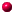´

Objects in SYMMETRICA

Symmetrica was written in the programming language C. The design of the routines was in a object oriented way.

In Symmetrica there is only one type of object. One component of the object is a tag identifying the specific kind of object. Using this tag the (meta-) routines (e.g. mult) switch to the specific routine (e.g. mult_permutation).

The advantage of this method is that we only have one routine for different kind of objects. So is the routine for the inversion of a matrix independent of the objects inside the matrix. We only need a routine to invert the entries.
But we have a overhead in the object to store the tag, and a overhead in runtime to switch to the specific routine. This method is simliar to virtual classes in C++.

Now a complete listing of available types of objects.INTEGERVECTOR of arbitray objectsBITVECTOR 0-1 vector as bit vectorPARTITIONBRUCH fraction of two arbitrary objectsPERMUTATIONSKEWPARTITIONTABLEAUXPOLYNOM polynomial with arbitrary coefficentsSCHUR Schur function with arbitrary coefficentsMATRIX of arbitray objectsHOMSYM complete symmetric functionSCHUBERT Schubert polynomialINTEGERVECTOR VECTOR object with INTEGER objectsINTEGERMATRIX MATRIX object with INTEGER objectsSYMCHAR charcter of the symmetric groupWORDLIST list of arbitray objectsLONGINTBINTREE binary tree of arbitray objectsPOWSYM symmetric power sumELMSYM elementary symmetric functionKRANZ element of wreath product of symmetric groupsFINITEFIELD element of a finite fieldGROUPALGEBRA element of the group algebra of a permutation groupREIHE seriesCYCLOTOMICMONOPOLY polynomial in one variableSQ_RADICALLAURENTBARPERM barred permutations

symmetrica (at) symmetrica.de

University of Bayreuth -